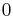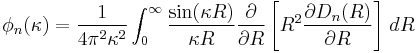# Editing Hints

### MATH$0$$\phi_n(\kappa) = \frac{1}{4\pi^2\kappa^2} \int_0^\infty \frac{\sin(\kappa R)}{\kappa R} \frac{\partial}{\partial R} \left [ R^2\frac{\partial D_n(R)}{\partial R} \right ] \,dR$

• name creates a link to the words to the right of the space after the link